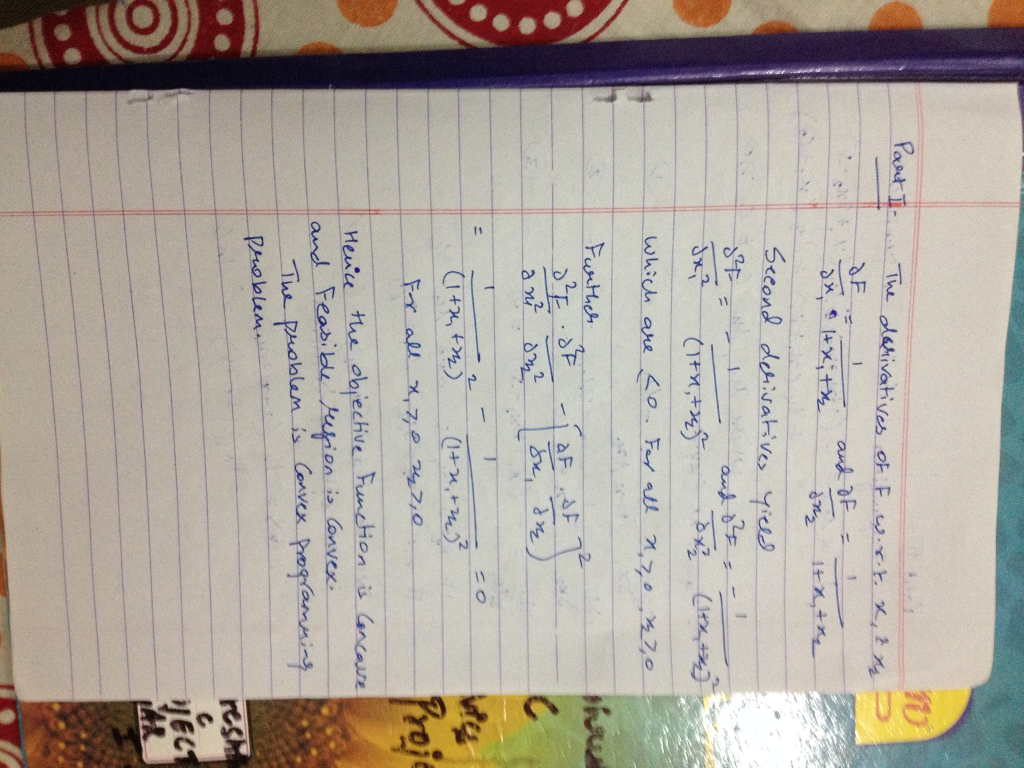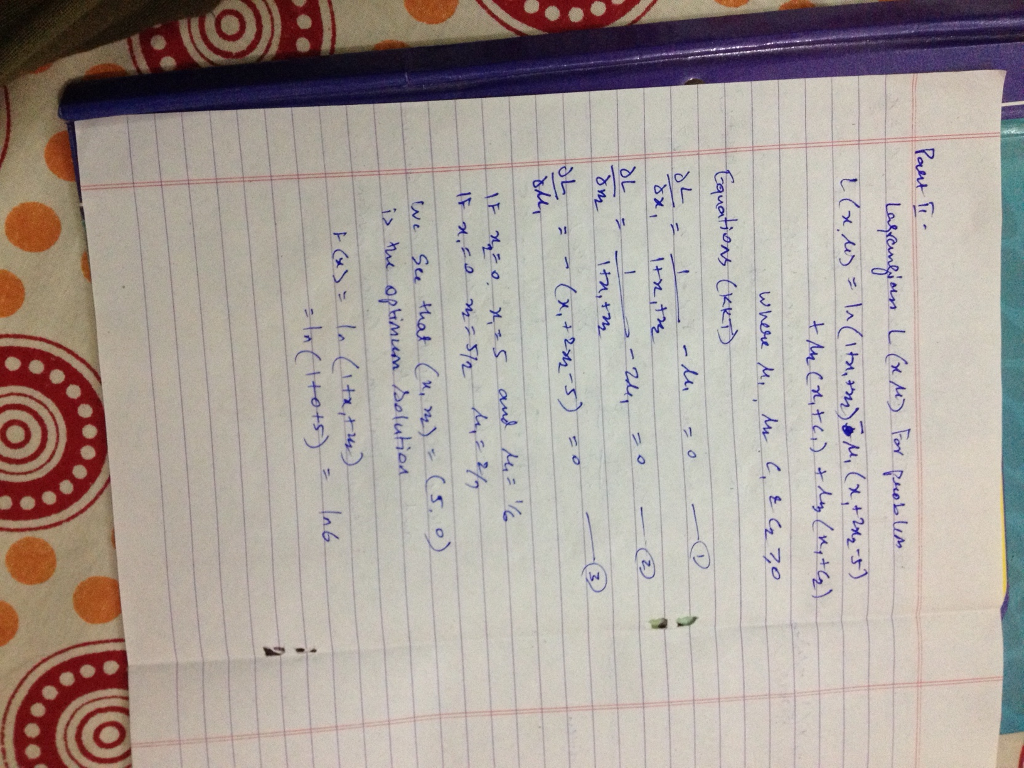# Solved: Consider the following linearly constrained optimization problem:

Consider the following linearly constrained optimization problem: Maximize f(x) = In(1 + x_1 + x_2), subject to x_1 + 2 x_2 lessthanorequalto 5 and x_1 greaterthanorequalto 0, x_2 greaterthanorequalto 0, where In denotes the natural logarithm. a) Verify that this problem is a convex programming problem. b) Use the KKT conditions to derive an optimal solution.